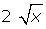## Exercise 1

Indicate which of the following expressions are monomials. If it is, indicate the degree and coefficient of the monomial. If it is not, state why it is not a monomial:

13x³

25x−3

33x + 1

4567## Exercise 2

Simplify:

12x²y³z + 3x²y³z

22x³ − 5x³ =

33x4 − 2x4 + 7x4 =

42 a²bc³ − 5a²bc³ + 3a²bc³ − 2 a²bc³ =

## Exercise 3

Solve:

1(2x³) · (5x³) =

2(12x³) · (4x) =

35 · (2x²y³z) =

4(5x²y³z) · (2y²z²) =

5(18x³y²z5) · (6x³yz²) =

6(−2x³) · (−5x) · (−3x²) =

## Exercise 4

Solve:

1(12x³) : (4x) =

2(18x6y²z5) : (6x³yz²) =

3(36x³y7z4) : (12x²y²) =

456## Exercise 5

Solve:

1(2x³)³ =

2(−3x²)³ =

3## Solution of exercise 1

Indicate which of the following expressions are monomials. If it is, indicate the degree and coefficient of the monomial. If it is not, state why it is not a monomial:

13x³

Degree: 3, coefficient: 3

2 5x−3

It is not a monomial because the exponent is not a natural number.

33x + 1

It is not a monomial because there is an addition.

4Degree: 1, coefficient:5Degree: 4, coefficient:6It is not a monomial because it does not have a natural exponent.

7It is not a monomial because the literal part is within a root.

## Solution of exercise 2

Simplify:

12x²y³z + 3x²y³z = 5x²y³z

22x³ − 5x³ = −3x³

33x4 − 2x4 + 7x4 = 8x4

42a²bc³ − 5a²bc³ + 3a²bc³ − 2a²bc³ = −2a²bc³

## Solution of exercise 3

Solve:

1(2x³) · (5x³) = 10x6

2(12x³) · (4x) = 48x4

35 · (2x² y³z) = 10x²y³z

4(5x²y³z) · (2 y²z²) = 10x²y5

5(18x³y²z5) · (6x³yz²) = 108x6y³z7

6(−2x³) · (−5x) · (−3x²) = −30x6

## Solution of exercise 4

Solve:

1(12x³) : (4x) = 3x²

2(18x6y²z5) : (6x³yz² ) = 3x³yz³

3(36x³y7z4) : (12x²y²) = 3xy5z4

454x³y + 3x²y² − 8x8

6## Solution of exercise 5

Solve:

1(2x³)³ = 2³ · (x³)³ = 8x9

2(-3x²)³ = (-3)³ · (x³)² = −27x6

3Did you like the article?(1 votes, average: 5.00 out of 5)Loading...

Emma

I am passionate about travelling and currently live and work in Paris. I like to spend my time reading, gardening, running, learning languages and exploring new places.

Did you like
this resource?

Bravo!